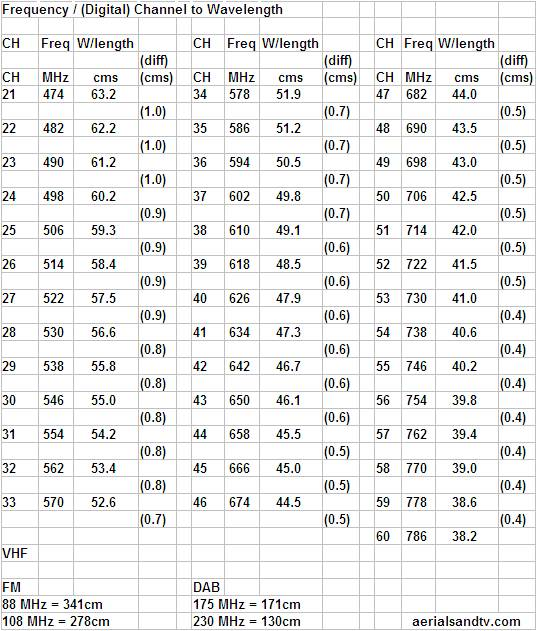## UHF channel numbers

In the UK television transmissions are usually referred to by a channel number (not to be confused with programme channel, e.g. BBC1) as opposed to a frequency. Both the channel number and the frequency are given on all our Channel Allocation Guides (e.g. the one for Crystal Palace), plus the accompanying table also shows channel number v frequency (and also the wavelengths).For digital the channel number is related to the frequency thus : Fc = 8n + 306
(where Fc = Centre frequency of the MUX, and n = Channel Number).

In the case of analogue the channel number is/was related to the frequency thus : Fv = 8n + 303.25
(where Fv = Frequency of the vision carrier in MHz and n = Channel Number).

Note analogue does still exist for modulated RF signal distribution !

As an approximation, good enough for most tuners, the analogue channel frequencies are 3MHz lower than the analogue (although it's actually 306 - 303.25 = 2.75MHz).

Since most tuners use channel numbers this 3MHz difference is not usually significant, but if you are trying to enter the actual frequency to yours it obviously might be for you ! Some digital MUXES are transmitted with a channel offset and these are + or - 0.167 MHz.The vast majority of digital TVs or STBs can still tune in to a MUX even if it has an offset and this is generally also the case in manual tuning. But if you have trouble trying to tune in your MUXES its always worth checking that the tuner is not being sidelined by an offset, though this should be rare.

## UHF (TV) & VHF (FM & DAB) frequency wavelengths

Wavelength = Velocity ÷ Frequency.
Speed of light = 299,792,458 m/s.

To calculate the wavelength (in cms) divide 30,000 by the frequency (in MHz).
This should be accurate to 0.07%, which is accurate enough for most RF applications...

The frequencies given in the table are for (most) digital MUXES, for analogue channels deduct 3MHz (see article above).

If cutting an FM Half Wave down to peak it on a frequency (or maximise DAB at the expense of FM) work out the wavelength, then divide by 4*, and cut each element to that length.
When we experimentally peaked up an FM Half Wave for DAB : the mid point frequency would be about 200MHz, the wavelength of that is 150cm (see above), divided by 4 is about 38cm (per element). And sure enough the DAB response did improve to just behind a DAB dipole, though the FM dropped off quite a way!
Similarly, to peak up the Half Wave to (say) 104MHz (wavelength 288cm) cut each element to 72cm. How much difference that would make in reception I'd be interested to know!

* It's a Half Wave dipole (i.e. divide by 2) and there are two elements making up the aerial (divide by 2 again).

## RF frequency bands

The terms aren't used so much now, but the RF spectrum used to be divided into “bands”. They were as follows, more or less anyway, the precise bands tended to vary throughout the world.

Band I = 47 to 88 MHz
Band II = 88 to 108 MHz (= approx UK FM band)
Band III = 174 to 240 MHz  (= approx UK DAB band)
Band IV = 470 to 614 MHz (= approx bottom half of the original UK TV band 21 to 38)
Band V = 614 to 854 MHz  (= approx top half of the original UK TV band 39 to 68)

Not specifically an RF frequency band bit of (almost) relevant interest :
Frequencies 700MHz to 4.2GHz used for Mobile and wireless broadband (as at Jan 2022)